085-1263404

### My Journal

All things Mathematical
1 Dec 2018

Special Thanks: A copy of this Leaving Cert Applied Maths Higher Level 1971 exam was kindly provided by Noel Cunningham.

## Question 1

a) Explain how a graph of velocity plotted against time can be used to calculate acceleration and distance travelled, with particular reference to motion with constant acceleration.

[Video Solution]

b) A pigeon in flight releases a small stone from its beak at a height of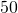metres when its velocity is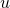. If the stone takes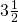seconds to reach the ground, show that the direction ofis not horizontal and compute the greatest height reached by the stone after release. (Give your answer correct to the nearest tenth of a metre.)

[Video Solution]

## Question 2

A particle is projected from a point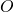on a plane inclined at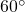to the horizontal with a velocity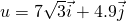metres/second where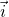is a unit vector throughpointing upward along the line of greatest slope in the plane and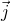is a unit vector perpendicular to the plane.

i) Show that after timeseconds the position vector,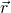of the particle relative tois given by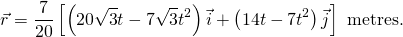ii) Prove that the range on the inclined plane is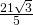metres,

iii) and find the velocity of the particle when it strikes the plane.

[Video Solution]

## Question 3

Two smooth spheres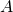and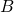of equal radii but of masses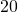kg and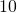kg respectively collide on a smooth horizontal table. Before collision the velocity ofis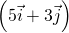m/s and the velocity ofis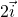m/s whereandare unit perpendicular vectors in the plane of the table andlies along the line of centres at impact. If the collision is perfectly elastic find the velocity ofand the velocity ofimmediately afterwards.

[Video Solution]

## Question 4

A light inextensible string which is fastened at one end to a point in the ceiling passes under a smooth movable pulleyof mass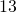kg, then over a smooth fixed pulley, and a particle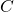of mass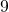kg hangs freely from its other side. All parts of the string which are not touching the pulleys are vertical.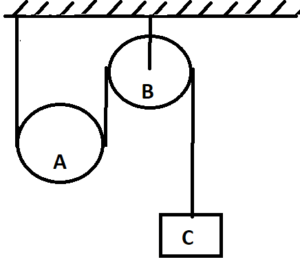i) When the system is released from rest show that the acceleration of the particle is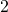m/sand that the acceleration of the pulley is half this.

ii) Find also the tension in the string.

[Video Solution]

## Question 5

a) Define simple harmonic motion in a straight line and show it can be described by the differential equation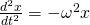. Prove that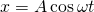, whereis a constant, is a solution of this equation.

[Video Solution]

b) A particle describing simple harmonic motion on a straight line has velocities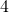m/s andm/s when at a distance ofm andm respectively from the centre of oscillation.

i) Find the amplitude and periodic time of the motion.

ii) Calculate the least time taken for the particle to travel from the position of rest to the point where the velocity wasm/s.

[Video Solution]

## Question 6

a) i) Show that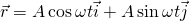, where,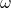are constants and,are constant unit perpendicular vectors, is the position vector at timeof a particle moving in a circle.

ii) Show that the velocity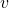is of magnitude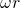and is at right angles to.

iii) Prove that the acceleration is of magnitude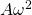and acts towards the centre of the circle.

[Video Solution]

b) A particle of masskg is describing a circle on a smooth horizontal table. It is connected by a light inelastic string of length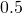m to a point which is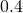m vertically above the centre of the circle. If the reaction of the table on the mass is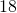N, calculate:

i) the constant angular velocity of the particle,

(ii) the tension of the string.

[Video Solution]

## Question 7

a) Two rods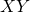,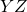, of equal lengths but of weights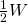and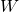, respectively, are freely hinged together at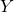. They stand in equilibrium in a vertical plane with the ends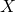and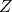on a rough horizontal plane with the angle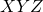equal to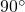.

i) Show that the vertical components of the action of the plane atandare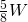and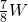respectively.

ii) Show in a separate diagram the forces acting on each rod, using vertical and horizontal components for the reaction at. Find all these forces and prove that if one rod is on the point of slipping, the coefficient of friction is.

[Video Solution]

## Question 8

i) Prove that the moment of inertia of a uniform circular lamina of mass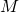and radius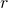about an axis  through its centre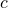, perpendicular to the plane of the lamina, is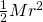.

[Video Solution]

ii) Deduce that the moment of inertia about a parallel axis through a point of the circumference is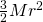.

[Video Solution]

iii) Such a lamina is free to rotate in a vertical plane about a horizontal axis perpendicular to its plane through a point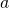on its circumference. It is released from rest with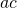horizontal. Find the angular velocity of the lamina wheremakes an angle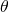with the download vertical, show that the speed ofat its lower point is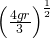.

[Video Solution]

## Question 9

a ) State Archimedes Principle for a body immersed in a liquid.

[Video Solution]

b) A uniform rod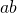in equilibrium is inclined to the vertical with one quarter of its length immersed under water and its upper endsupported by a vertical force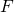.

i) Show that in a diagram the three force acting on the rod, and prove that the specific gravity of the rod is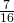.

ii) Expressin terms of, the weight of the rod.

[Video Solution]

## Question 10

A particle of massis projected with speedalong a smooth horizontal table. The air resistance to motion when the speed of the particle is, is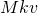where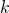is a constant.

i) By solving the equation of motion for the particle, show that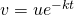and

ii) prove that as time increases indefinitely, the distance travelled ultimately approaches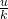.

iii) Find the time taken for the particle to travel half this distance.

[Video Solution]

### Latest PSI Licence:

“Contains Irish Public Sector Information licensed under a Creative Commons Attribution 4.0 International (CC BY 4.0) licence”.

### Important Exception to the above Licence:

The State Examination Commission is the copyright holder which is providing the material under the above license (as per current directives and regulations from the relevant government bodies). However the State Examination Commission as an Irish examination body is able to use copyrighted material in its exams without infringing copyright but this right is not extended to third parties when those exams are re-used.

(For example: the State Examination Commission may include in their exam a copyrighted poem and this action does not require the permission of the poet but the poet’s permission must be sought when the exam is re-used by someone other than the State Examination Commission.)

Also, all derived and related work (such as video solutions, lessons, notes etc) are the copyrighted material of Stephen Easley-Walsh (unless stated otherwise). And that the above licence is for only the exam itself and nothing further.

### Citation:

State Examinations Commission (2018). State Examination Commission. Accessed at: https://www.examinations.ie/?l=en&mc=au&sc=ru

Malone, D and Murray, H. (2016). Archive of Maths State Exams Papers. Accessed at: http://archive.maths.nuim.ie/staff/dmalone/StateExamPapers/

This site uses Akismet to reduce spam. Learn how your comment data is processed.